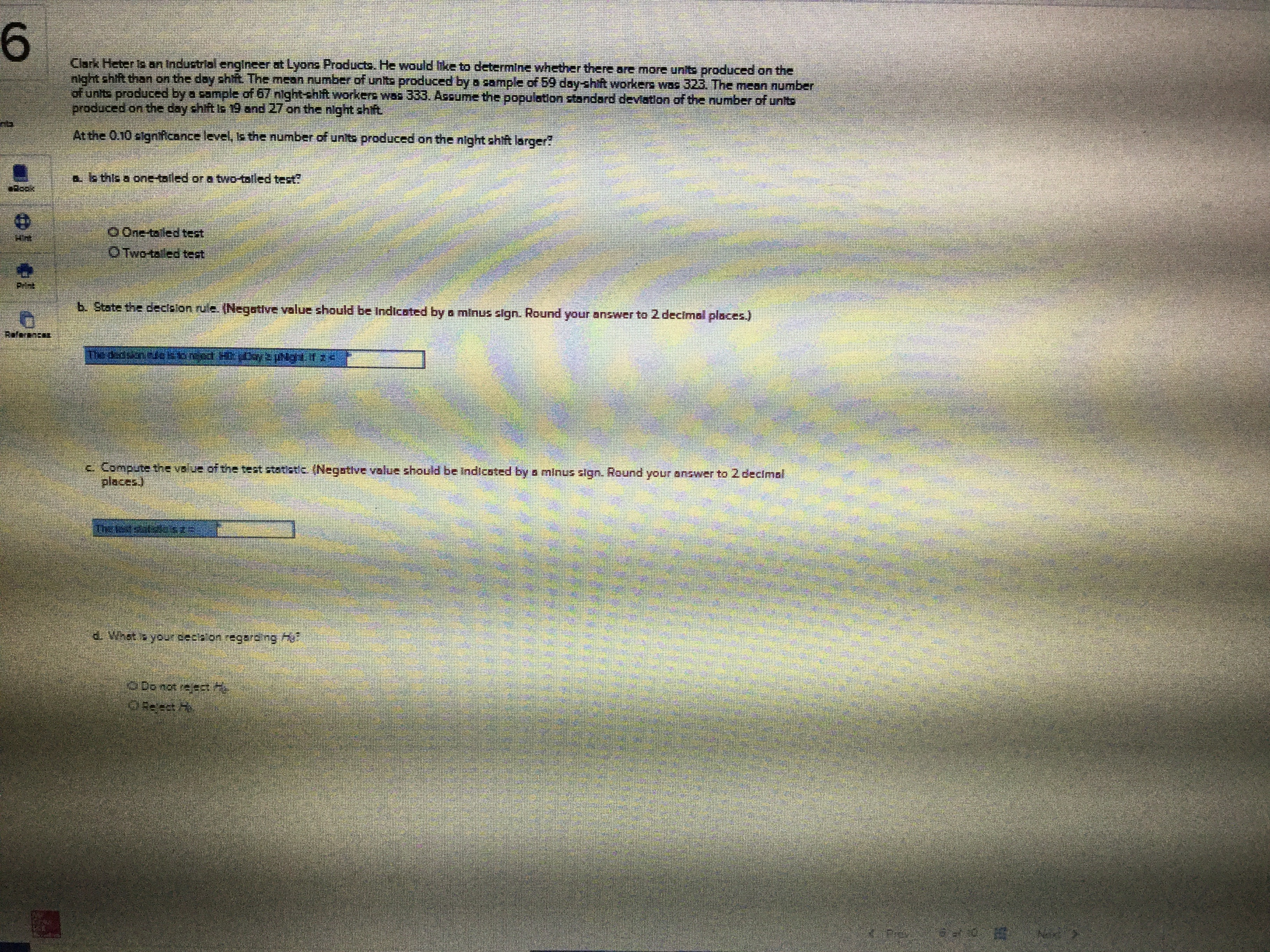# 6Clark Heter is an Industrial engineer at Lyons Products. He would ike to determine whether there are more units produced on thenight shift than on the day shtf The mean number of units produced by a sample of 59 day-hft workers was 323. The mean numberof units produced by a sample of 67 night-shit workers wos 333. Assume the population stondard deviaton of the number of unitsproduced on the day shtft Is 19 and 27 on the nilght shiftAt the 0.10 slgnffcance level, is the number of units produced on the night shift larger?&. s this a one-tailed or a two-talled test?OOne taled testOTwo-taled testb. State the decialon rule. (Negative value should be Indicated by a minus stgn. Round your answer to 2 decimal places)RufarKc Compute the value of the test statatic (Negative value should be Indicsted by s minus slgn. Round your answer to 2 decimalplaces)The estzsszDo not rejesh

Question
34 views

I wwould like the solutions for parts a-dhelp_outlineImage Transcriptionclose6 Clark Heter is an Industrial engineer at Lyons Products. He would ike to determine whether there are more units produced on the night shift than on the day shtf The mean number of units produced by a sample of 59 day-hft workers was 323. The mean number of units produced by a sample of 67 night-shit workers wos 333. Assume the population stondard deviaton of the number of units produced on the day shtft Is 19 and 27 on the nilght shift At the 0.10 slgnffcance level, is the number of units produced on the night shift larger? &. s this a one-tailed or a two-talled test? OOne taled test OTwo-taled test b. State the decialon rule. (Negative value should be Indicated by a minus stgn. Round your answer to 2 decimal places) RufarK c Compute the value of the test statatic (Negative value should be Indicsted by s minus slgn. Round your answer to 2 decimal places) The estzssz Do not rejesh fullscreen
check_circle

Step 1

The provided information’s are:

Sample size (n1) = 59

Sample mean of night shift = 323

Standard deviation (σ1) = 19

Sample size (n2) = 67

Sample mean of day shift = 333

Standard deviation (σ2) = 27.

Significance level (α) = 0.01

Step 2

The null and alternative hypotheses are:

Step 3

The test is one sided that is right tail.

The t...

### Want to see the full answer?

See Solution

#### Want to see this answer and more?

Solutions are written by subject experts who are available 24/7. Questions are typically answered within 1 hour.*

See Solution
*Response times may vary by subject and question.
Tagged in

### Statistics